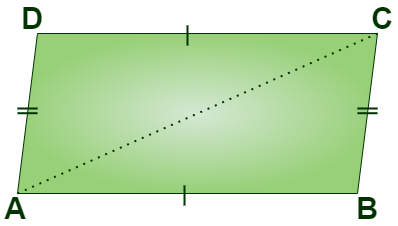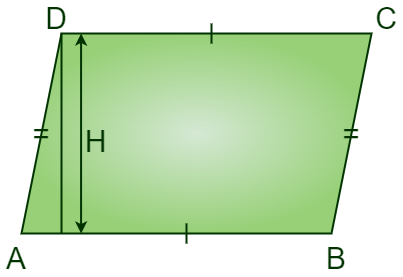GeeksforGeeks App
Open AppBrowser
Continue

# Height of a Parallelogram Formula

Height of a parallelogram formula is helpful in calculating height of a parallelogram. The height of a parallelogram is the perpendicular distance between the base side and the parallel side opposite to it.

A parallelogram can be defined as a quadrilateral in which opposite sides are parallel and the length of these opposite sides is also equal. Its opposite angles are also equal.## What is the Height of a Parallelogram Formula?

Height of a parallelogram is calculated using the formula Area is equal to Base multiplied by Height, so if the area of a parallelogram is known its height is calculated by dividing the Area by its Base.## Height of a Parallelogram Formula

The height of a parallelogram formula is derived from the area formula, area of a Parallelogram can be found using the base length and height of the parallelogram.

Area = Base × Height

Height = Area/Base

### Perimeter of a parallelogram

Perimeter is defined as the sum of all sides using which a closed figure is been constructed. In the case of a parallelogram, it has 4 sides out of which opposite sides are equal so the perimeter is the sum of all four sides.

Let’s take the measure of opposite sides as X and Y hence

Perimeter of Parallelogram = X + Y + X + Y = 2X + 2Y = 2(X + Y)

Perimeter = 2(X + Y)

## Solved Example on Height of Parallelogram

Example 1: Find the height of a parallelogram if its area is 625 cm2 and the length of the base is 25 cm.

Solution:

The height of a parallelogram can be calculated using the height of a parallelogram formula

Height = Area /Base

Height = 625/25

Height = 25 cm.

Hence the height of the parallelogram is 25 cm

Example 2: Find the area of a parallelogram whose base is 20 cm and height is 15 cm.

Solution:

Area of parallelogram = Base × Height

Base and height are given as 20 cm and 15 cm respectively.

Area = 20 × 15

Area = 300 cm.

Example 3: Perimeter of a parallelogram is 226 cm and one side of the parallelogram is of length 12 cm, find the measure of the other side.

Solution:

Perimeter of Parallelogram = 2(X + Y)

Where X is length of one side and Y is length of other side, so let X = 12 cm

226 = 2(X + Y)

2(X + Y) = 226

2(12 + Y) = 226

24 + 2Y =226

2Y = 226 – 24

2Y = 202

Y = 202/2

Y = 101 cm.

Example 4: Find the height of a parallelogram if its area is 800 cm2 and the length of the base is 40 cm.

Solution

The height of a parallelogram can be calculated using the height of a parallelogram formula

Height = Area /Base

Height = 800/40

Height = 20 cm.

Hence the height of the parallelogram is 20 cm

Example 5: Perimeter of a parallelogram is 400 cm and one side of the parallelogram is of length 80 cm, find the measure of another side.

Solution:

Perimeter of Parallelogram = 2(X + Y)

Where X is length of one side and Y is length of other side, so let X = 80 cm

400 = 2(X + Y)

2(X + Y) = 400

2(80 + Y) = 400

160 + 2Y =400

2Y = 400 – 160

2Y = 240

Y = 120 cm.

## FAQs on Height of Parallelogram

Question 1: What is the base and height of a parallelogram?

“Base” refers to the length of a side and “Height” is the length of a perpendicular between two parallel lines.

Question 2: What is Height of a Parallelogram?